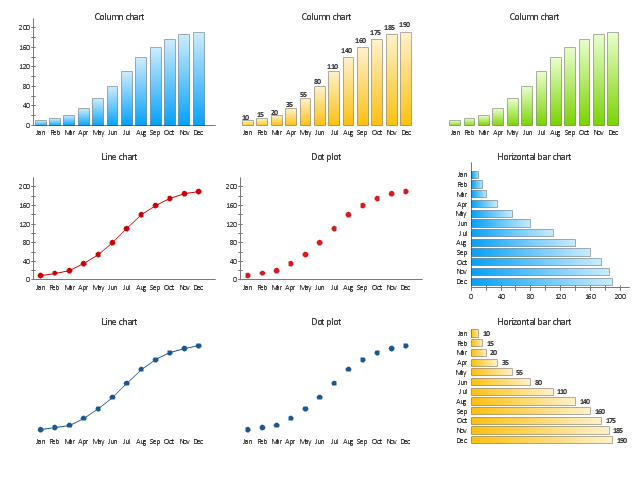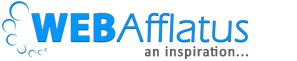# Exporting multiple graphs in same plot to PDF in R – TopBullets.comAs being a data scientist, plotting data is one of the first things we generally do. Without studying the behavior of the data we can’t or rather should not move ahead. There can be a lot of analysis which we can perform by plotting the graphs for example univariate, bivariate and residual plots. In my earlier blog, I wrote about how to plot two graphs in the same plot using par () function in R which is very useful when we do bivariate analysis and want to see the behavior of 2 variables across different time duration. Today I will write how to export the plots in PDF and in a tabular format. Generally exporting plots in any format (JPG, PDF) is an easier task but when you have say 50 graphs, you won’t want 50 pages, rather you will want 4 graphs in one page which will be very easier to read or interpret.

```################################################################
################### R Tutorial ###############################

# Purpose: Plot multiple graphs and export to PDF
# Author: Deepesh Singh

################################################################

#Creating random dataset
set.seed(199123)

# Creating random population by year
men <- seq (10, 22, by = ((22-10)/(35 - 1)))
women <- seq (20, 32, by = ((32-20)/(35 - 1)))
income <- sample(25, size = 35, replace = T)
birthrate <- sample(15, size = 35, replace = T)
car_purchase <- sample(10, size = 35, replace = T)

year <- seq(1982,2016)

# Checking the length of each variable to create a dataframe
length(men)
length(income)
length(year)
length(women)
length(birthrate)
length(car_purchase)

# Merging them all to form data frame
india_demo <- data.frame(year,men,women,income,birthrate, car_purchase)

###########################################################
######## Plotting the graphs ###########################
# Now we want to plot men population against time and want to check
# the correlation with other variables

# how many variables I should plot
no_col <- ncol(india_demo) - 2 # -2 because year will be constant X axis and men will be constant Y1
no_rows <- ceiling(no_col/2) # /2 because I want 2 plots in 1 row

# Creating new PDF file
pdf("Export_Plots.pdf", width = 16 , height = 10, title = "EDA Plots for data")

# Deciding rows and cols
par(mfrow=c(2,2))

for(i in 3:(ncol(india_demo))){

# Setting margin
par(mar = c(5,4,4,5)+.1)
# plotting primary Y axis which is always "men"
plot(india_demo[,1], india_demo[,2], col = "black", type = "l", ylab = names(india_demo), xlab = "Year")
par(new = T)

# Plotting secondary Y axis
plot(india_demo[,1], india_demo[,i], col = "red", type = "l", xaxt = "n", yaxt = "n",
xlab = "", ylab = "")
axis(4)
mtext(names(india_demo[i]), side = 4, line = 3)

}

dev.off()

```

I think the below code is self-explanatory but if you have any further doubt please do comment below.
Useful tags:
1. Error in plot.new() : figure margins too large
2. Export graphs to PDF, JPG in R
3. Multiple graphs in the same plot
4. Plotting graph on secondary axis in R

##### Signature

Deepesh Singh